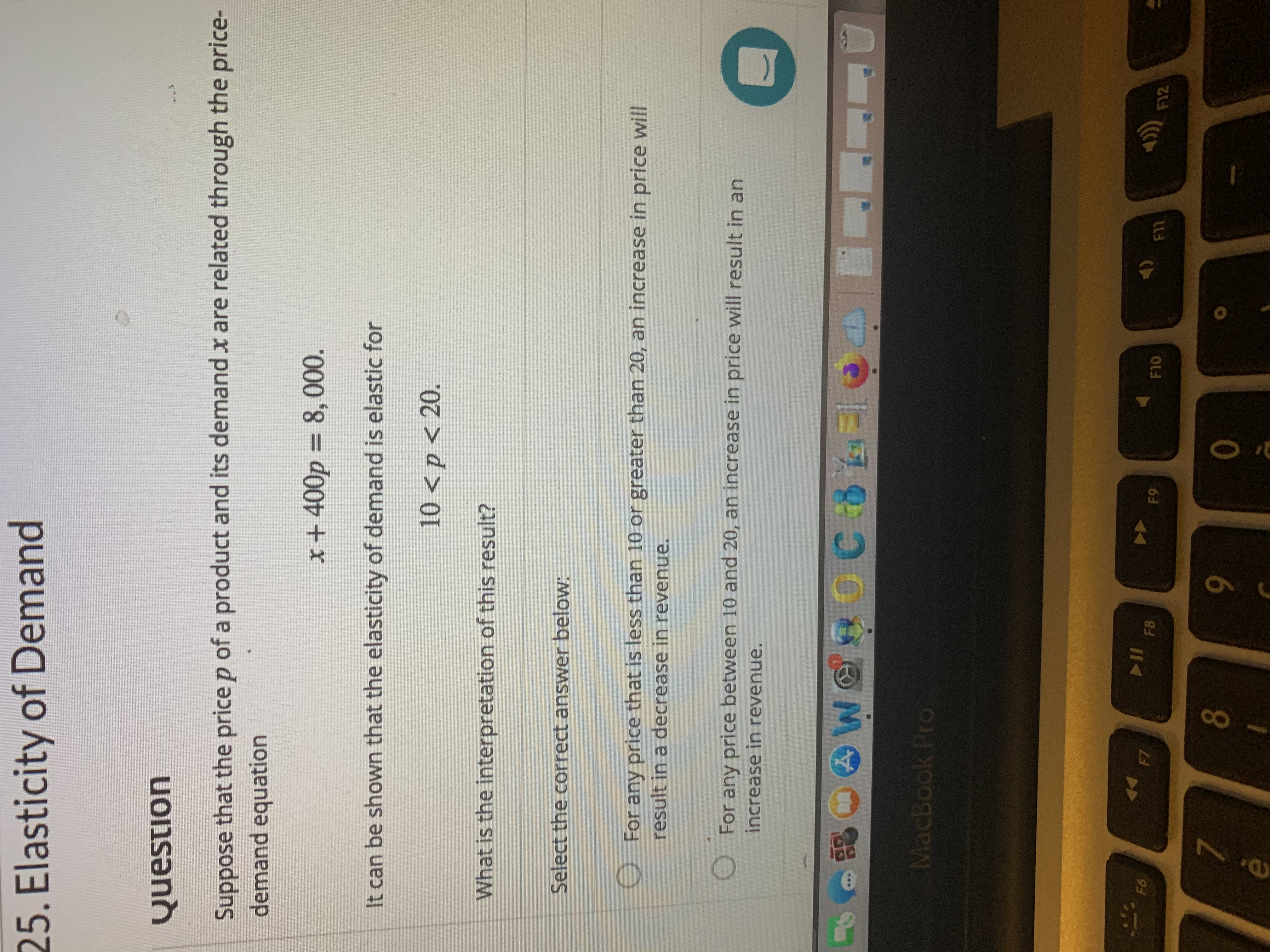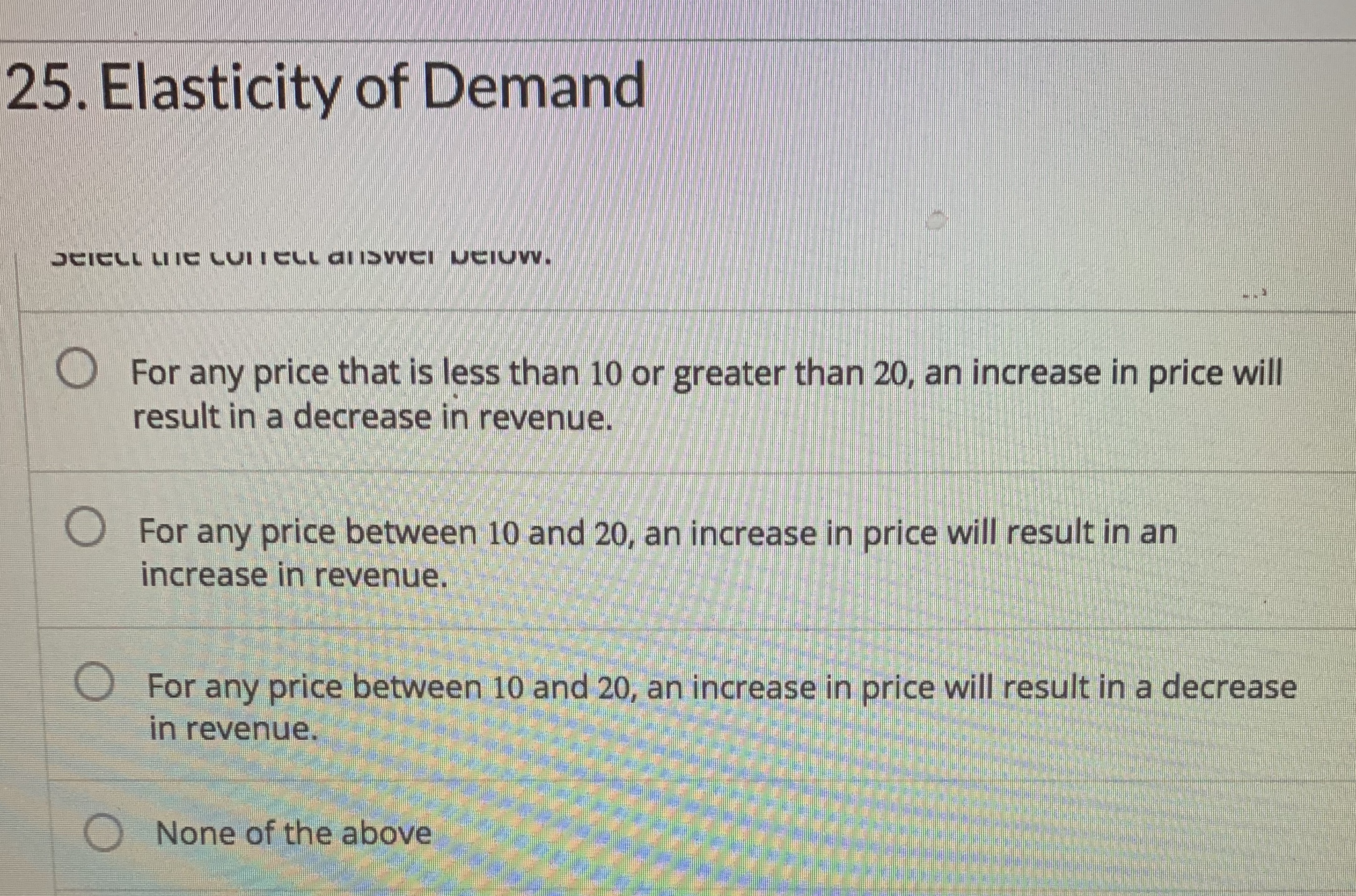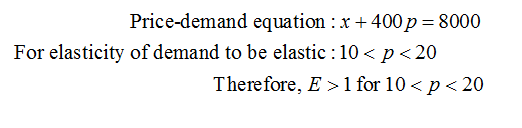# 25. Elasticity of DemandQuestionSuppose that the price p of a product and its demand x are related through the price-demand equationx+400p = 8, 000.It can be shown that the elasticity of demand is elastic for10

Question
2 viewshelp_outlineImage Transcriptionclose25. Elasticity of Demand Question Suppose that the price p of a product and its demand x are related through the price- demand equation x+400p = 8, 000. It can be shown that the elasticity of demand is elastic for 10help_outlineImage Transcriptionclose25. Elasticity of Demand UAICLL LIIE CUITELL aliSvVEI UCIUW. O For any price that is less than 10 or greater than 20, an increase in price will result in a decrease in revenue. O For any price between 10 and 20, an increase in price will result in an increase in revenue. O For any price between 10 and 20, an increase in price will result in a decrease in revenue. O None of the above fullscreen
check_circle

Step 1

Third option is the correct option.

Consider given price demand equation and the condition for the elasticity of the demand....

### Want to see the full answer?

See Solution

#### Want to see this answer and more?

Solutions are written by subject experts who are available 24/7. Questions are typically answered within 1 hour.*

See Solution
*Response times may vary by subject and question.
Tagged in